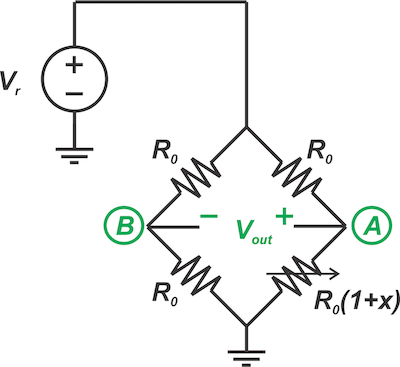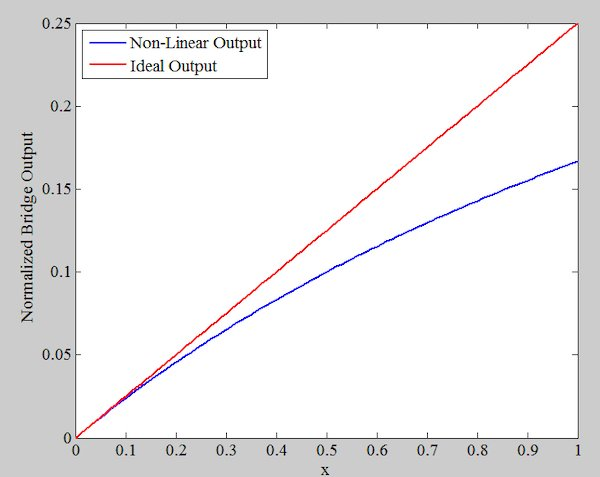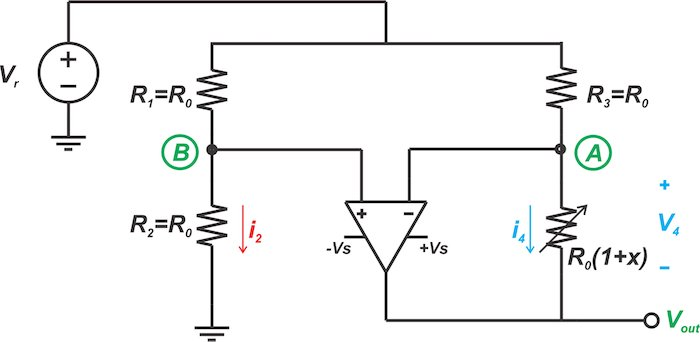The resistance of a resistive sensor depends on a physical variable such as temperature or force. The percentage change in the resistance of these devices is usually small. For example, the total change in the resistance of a strain gauge might be less than 1% over its entire operating range.

Discerning these small values mandates highly accurate measurement circuits. Bridge circuits allow us to more easily perform these accurate measurements. However, the output of a bridge circuit might have a non-linear relationship with the measured physical variable even if we are using a linear sensor.

In these cases, we can use either software or hardware techniques to eliminate the bridge non-linearity errors. In this article, we’ll take a look at two different techniques of linearizing resistive sensor bridges.

### Bridge Non-linearity of Resistive Sensors

Consider a resistive pressure sensor with the following linear response:

$R_{sensor} = R_0 + M_x$

where R0 is the initial resistance of the sensor at zero pressure, x is the value of the measure and (pressure), and M is the slope of the sensor response. To make our future equations simpler, let’s assume that the value of M is equal to the value of the sensor’s initial resistance (R0) and hence, the sensor response is R0(1+x)

Usually, the percentage change in the resistance of a resistive sensor is small and we need to employ a bridge circuit to more easily perform accurate measurements. A common bridge configuration for this sensor is depicted in Figure 1.##### Figure 1. Common bridge configuration of a resistive sensor

Note that the other three resistors of the bridge have a resistance of R0. This choice of bridge resistors maximizes the sensitivity of the output (Vout) to changes in the sensor resistance. The output equation can be obtained as:

$V_{out} = V_A – V_B = V_r\left(\frac{R_0(1+x)}{R_0+R_0(1+x)} – \frac{1}{2}\right)$

This simplifies to:

$V_{out} = V_r\left(\frac{x}{2(2+x)}\right)$

##### Equation 1.

As you can see, the relationship between the bridge output and the change in the resistance value (x) is not linear. With $x\ll2$, we can approximate the above equation by the following linear relationship:

$V_{out} \approx V_r\left(\frac{x}{4}\right)$

##### Equation 2.

Figure 2 depicts the normalized output of the bridge VoutVr for both the actual case (Equation 1) and the ideal output (Equation 2).##### Figure 2. Non-linear (blue) and ideal (red) outputs of Equations 1 and 2

As expected, the deviation from the linear response increases with x.

### How Much Non-linearity Error Will Be Introduced?

Let’s quantify the non-linearity error of the above bridge circuit. We can rewrite Equation 1 as:

$V_{out} = V_r \left(\frac{x}{4}\right) \left(\frac{1}{1+ \frac{x}{2}}\right)$

Assuming that x21, we can use Taylor’s theorem to obtain an approximation of the above function as:

$V_{out} = V_r\left(\frac{x}{4}\right)\left(1 – \frac{x}{2}\right)$

Comparing this result with Equation 2, we can calculate the magnitude of the error as:

$E_{Non-Linearity} = V_r\left(\frac{x}{4}\right)\left(\frac{x}{2}\right)$

Dividing this to the expected ideal value given by Equation 2, we can obtain the percentage end-point linearity error for a given change in resistance (x):

$Percentage~Error = \frac{x}{2} \times 100\%$

#### Example of Finding a Linear Response

Consider a sensor with response Rsensor = R0(1 + x). Assume that R0 = 100 Ω and the maximum value of x over the entire operating range is 0.01. The maximum linearity error percentage will be:

$Percentage~Error = \left(\frac{0.01}{2}\right) \times 100\% = 0.5\%$

Note that although we might be able to use software to remove the sensor linearity errors, having a linear response is desirable since it increases measurement precision and facilitates system calibration. There are different circuit topologies that can be used to linearize bridge circuits.

In the rest of this article, we’ll examine two different bridge linearization techniques.

### Method 1: Finding the Voltage of a Linear Sensor With a Fixed Current

The first linearization technique that we’ll discuss in this article is shown in Figure 3. Let’s first examine the basic idea of this technique and then see how the circuit in Figure 3 implements this idea.##### Figure 3. One type of circuit for linearizing resistive sensor bridges

Figure 4 shows a depiction of a fixed current of IRef forced to flow through our linear sensor.##### Figure 4. A fixed current (IRef) forced through a linear sensor

In this scenario, the resulting voltage across the sensor would be:

$V_{sensor} = I_{Ref} \times R_0(1 + x)$

that can be rearranged as:

$V_{sensor} = R_0 \times I_{Ref} + R_0 \times I_{Ref} \times x$

While the first term is a constant value, the second term is proportional to the changes in the sensor resistance (x). If we can omit the constant term, we’ll have a voltage that has a linear relationship with x.

#### Circuit Implementation

The circuit in Figure 3 uses the above idea to linearize the bridge circuit. Since the op-amp inputs ideally do not draw any current, the voltage at node B will have a constant value of:

$v_B = \frac{R_0}{R_0 + R_0}V_r = \frac{V_r}{2}$

The negative feedback along with the high gain of the op-amp will force both the inverting and non-inverting inputs of the op-amp to have the same voltage:

$v_A = v_B = \frac{V_r}{2}$

Since both ends of R3 are at constant potentials, a constant current will flow through it. In other words, the op-amp makes R3 act as a current source that forces a constant current of $\frac{V_r}{2R_0}$ into the sensor. Hence, the voltage across the sensor will be:

$V_4 = \frac{V_r}{2R_0} \times R_0(1 + x) = \frac{V_r}{2} + \frac{V_r}{2}x$

The first term is the constant value that should be eliminated from the Vout equation. The second term is proportional to the sensor resistance changes (x) and should appear in the output equation. Applying Kirchhoff’s voltage law, we find Vout as:

$V_{out} = -V_4 + V_A = – \left(\frac{V_r}{2} + \frac{V_r}{2}x\right) + V_A$

Hence, we only need VA to be equal to $\frac{V_r}{2}$. This is already satisfied, which leads to:

$V_{out} = -\frac{V_r}{2}x$

Therefore, the output has a linear relationship with x.

### Method 2: Forcing a Current Proportional to Sensor Resistance##### Figure 5. Another circuit for the analog linearization of resistive sensor bridges

Let’s again take a look at the basic idea of this technique and then examine its circuit implementation.

This second linearization technique is illustrated in Figure 6.##### Figure 6. Linearization technique forcing current through a branch of the circuit to be proportional to the sensor’s resistance

It forces the current through a branch of the circuit (Branch 1) to be proportional to the sensor resistance:

$I_1 = I_{Ref} \times R_0(1 + x)$

where IRef is a constant value. Then, it performs current domain subtraction to eliminate the constant term IRef x R0. To this end, the current through Branch 2 is set to be IRef x R0. Hence, the current through Branch 3 will be IRef x R0xproportional to the changes in the sensor resistance (x).

#### Circuit Implementation

Let’s see how the circuit in Figure 5 implements the above idea. Again, the negative feedback along with the high gain of the op-amps will force both the inverting and non-inverting inputs of the two op-amps (both A1 and A2) to have the same voltage:

$v_A = v_B = 0$

##### Equation 3.

Hence, we have V1 = V2 that leads to

$R_0 (1 + x) \times I_1 = R_0 \times I_2$

This simplifies to:

$I_2 = I_1 + I_1 \times x$

##### Equation 4.

We know that I1 = I4 and, taking Equation 3 into account, we have:

$I_1 = I_4 = \frac{V_r – v_A}{R_0} = \frac{V_r}{R_0}$

Substituting this into Equation 4, we obtain:

$I_2 = \frac{V_r}{R_0} + \frac{V_r}{R_0} \times x$

Hence, I2 is the sum of a constant value and a term that is proportional to x. We only need to use Kirchhoff’s current law to eliminate the constant term in the output current equation. The current through R2 sources a current equal to $\frac{V_r}{R_0}$ to node A, leading to:

$I_F = -\frac{V_r}{R_0} \times x$

Therefore, we obtain:

$V_{out} = V_r \times \frac{R_F}{R_0} \times x$

The circuit in Figure 5 needs an additional op-amp compared to the first technique. However, with the two op-amp solutions, we can arbitrarily set the gain by choosing the $\frac{R_F}{R_0}$ ratio.

This post was first published on: All About Circuits